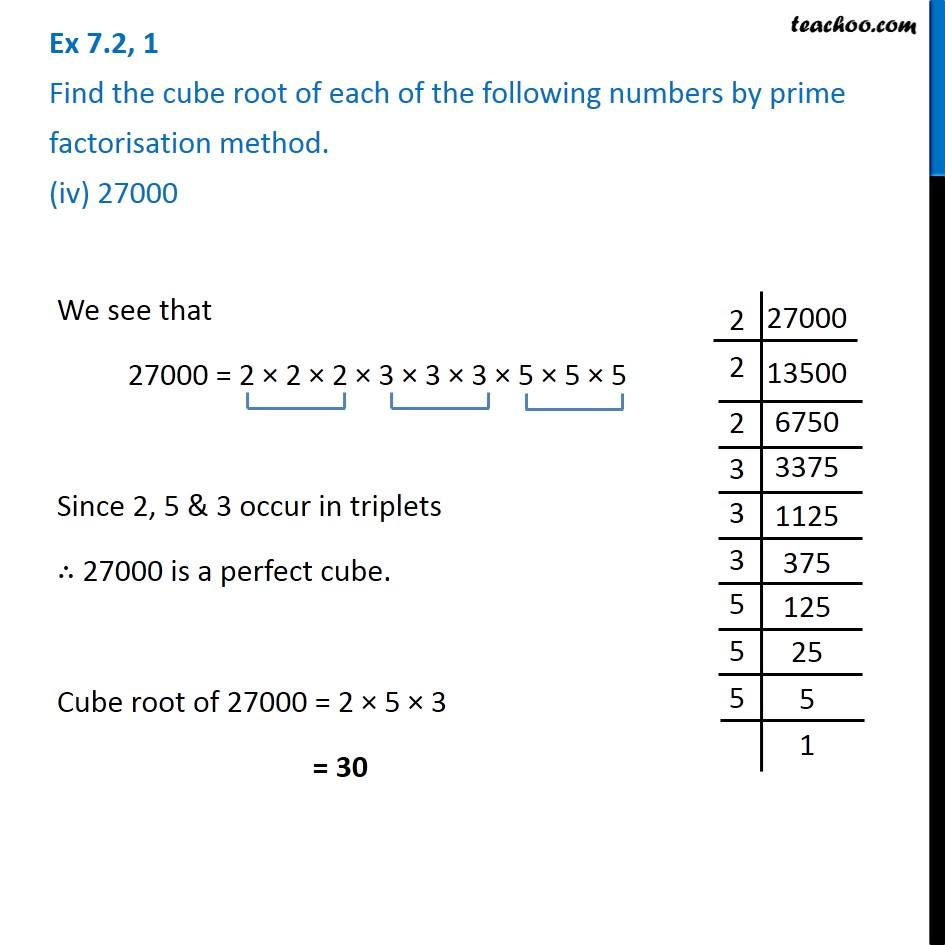1. Chapter 7 Class 8 Cubes and Cube Roots
2. Serial order wise
3. Ex 7.2

Transcript

Ex 7.2, 1 Find the cube root of each of the following numbers by prime factorisation method. (iv) 27000We see that 27000 = 2 × 2 × 2 × 3 × 3 × 3 × 5 × 5 × 5 Since 2, 5 & 3 occur in triplets ∴ 27000 is a perfect cube. Cube root of 27000 = 2 × 5 × 3 = 30